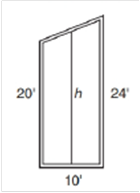Chapter 4.4, Problem 23EElementary Geometry For College St...

7th Edition
Alexander + 2 others
ISBN: 9781337614085

Solutions

Chapter
SectionElementary Geometry For College St...

7th Edition
Alexander + 2 others
ISBN: 9781337614085
Textbook Problem

Each vertical section of a suspension bridge is in the shape of a trapezoid. For additional support, a vertical cable is placed midway as shown. If the two vertical columns shown have heights of 20 ft and 24 ft and the section is 10 ft wide. What will the height of the cable be?To determine

To Find:

The height of the vertical cable.

Explanation

In the given problem, the vertical columns are parallel.

It becomes the bases of the trapezoid.

The mid cable acts like a median of the trapezoid.

Therefore, to find the height of the mid cable, find the median of the trapezoid.

It is given that the heights (bases of trapezoid) of the vertical columns are 20 ft. and 24 ft.

We know that, the length of the median of a trapezoid is the average of the lengths of the bases...

Still sussing out bartleby?

Check out a sample textbook solution.

See a sample solution

The Solution to Your Study Problems

Bartleby provides explanations to thousands of textbook problems written by our experts, many with advanced degrees!

Get Started

In Problems 27-30, write the equation of the tangent line to each curve at the given point. 27.

Mathematical Applications for the Management, Life, and Social Sciences

For the following set of scores, find the value of each expression: a. X b. (X)2 c. X2 d. (X +3)

Essentials of Statistics for The Behavioral Sciences (MindTap Course List)

cosh 0 = a) 0 b) 1 c) e22 d) is not defined

Study Guide for Stewart's Single Variable Calculus: Early Transcendentals, 8th